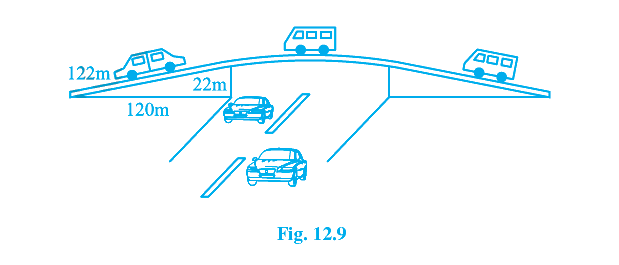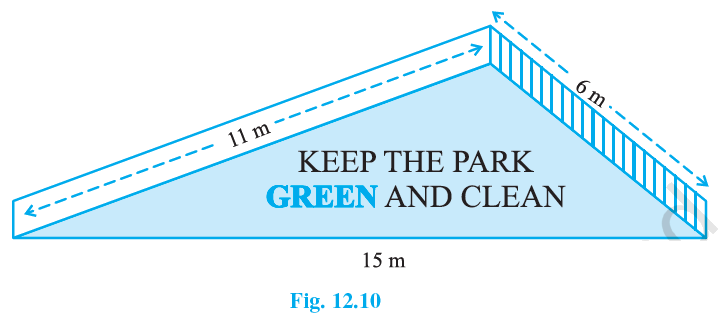NCERT Class 9 Maths Heron’s Formula

# NCERT Class 9 Maths Heron’s Formula

The chapter 12 begins with the general formula of area of a triangle involving base and height which leads to Heron’s formula involving the three sides of the triangle. Various examples are shown. The focus then shifts to applications of Heron’s formula in finding areas of the quadrilateral. Different examples are shown to demonstrate the same.

Download FREE PDF of Chapter-12 Heron’s Formula

## Chapter 12 Ex.12.1 Question 1

A traffic signal board, indicating ‘SCHOOL AHEAD’, is an equilateral triangle with side ‘$$a$$’. Find the area of the signal board, using Heron’s formula. If its perimeter is $$180 \;\rm{cm}$$, what will be the area of the signal board?

### Solution

What is known?

Dimensions of the traffic signal board (triangle) and its perimeter.

#### What is Unknown?

Area of the signal board.

Reasoning:

By using Heron’s formula we can calculate the area of triangle.

The formula given by Heron about the area of a triangle

\begin{align}=\sqrt{s(s-a)(s-b)(s-c)}\end{align}

Where $$a, b$$ and $$c$$ are the sides of the triangle, and

\begin{align}s &= \text{Semi-perimeter}\\& = \begin{Bmatrix} \text{Half the Perimeter } \\ \text{ of the triangle} \;\end{Bmatrix} \\&=\frac{(a+b+c)}{2}\end{align}

Steps:

Each side of traffic signal board (triangle)$$=‘a’ \;\rm{cm}$$

Perimeter of traffic signal board (triangle) $$=$$ sum of all the sides

\begin{align} &=a+a+a \\ &=3 a \end{align}

Semi Perimeter

\begin{align} s&=\frac{(a+b+c)}{2}\\&=\frac{a+a+a}{2}\\&=\frac{3 a}{2} \end{align}

By using Heron’s formula,

Area of a triangle

$$=\sqrt{s(s-a)(s-b)(s-c)}$$

Area of a (triangle) traffic signal board

\begin{align}&=\sqrt{s(s-a)(s-b)(s-c)} \\& =\sqrt{s(s-a)(s-a)(s-a)} \\& =(s-a) \sqrt{s(s-a)}\quad....(1)\end{align}

We know \begin{align}s=\frac{3 a}{2}\end{align} so substituting in equation (1)

\begin{align}&{=\left(\frac{3 a}{2}-a\right) \sqrt{\frac{3 a}{2}\left(\frac{3 a}{2}-a\right)}} \\ &{=\left(\frac{a}{2}\right) \sqrt{\frac{3 a}{2}\left(\frac{a}{2}\right)}} \\ &{=\frac{a}{2} \times \frac{a}{2} \sqrt{3}} \\ &{=\frac{a^{2}}{4} \sqrt{3}}\end{align}

Area of the signal board

\begin{align}=\frac{a^{2}}{4} \sqrt{3} \;\; \text{sq. units}\end{align}

Now given perimeter $$=$$ $$180 \;\rm{cm}$$

Each side of triangle

\begin{align}&=\frac{{180}}{3}\,\mathrm{cm} \\ a &={60 \,\mathrm{cm}}\end{align}

Area of the signal board

\begin{align}&={\frac{a^{2}}{4} \sqrt{3}} \\ &={\frac{60^{2}}{4} \sqrt{3}}\end{align}

Area of the signal board

$$=900 \sqrt{3} \;\rm{cm^2}$$

## Chapter 12 Ex.12.1 Question 2

The triangular side walls of a flyover have been used for advertisements. The sides of the walls are $$122$$ m, $$22$$m and $$120$$m see Figure. The advertisements yield an earning of Rs $$5000$$ per sq. m per year. A company hired one of its walls for $$3$$ months. How much rent did it pay?### Solution

What is known?

Dimensions of the triangular sides of walls.

What is Unknown?

Rent to pay.

Reasoning:

By using Heron’s formula we can calculate the area of triangle.

The formula given by Heron about the area of a triangle

$$=\sqrt{s(s-a)(s-b)(s-c)}$$

Where $$a, b$$ and $$c$$ are the sides of the triangle, and

\begin{align}s &= \text{Semi-perimeter} \\ & = \begin{Bmatrix} \text{ Half the Perimeter } \\ \text{of the triangle} \end{Bmatrix} \\ & =\frac{(a+b+c)}{2}\end{align}

Steps:

Triangular sides of walls are:

$$a= 122 \;{\rm{m}} , b = 22 \;{\rm{m}}, c = 120\;\rm{ m}$$

Semi Perimeter

\begin{align}s &=\frac{(a+b+c)}{2} \\ &=\frac{122+22+120}{2} \\ &=\frac{264}{2} \\&=132 \; \rm{m}\end{align}

By using Heron’s formula,

Area of a triangle

$$=\sqrt{s(s-a)(s-b)(s-c)}$$

Area of triangular wall

\begin{align}&=\sqrt{s(s-a)(s-b)(s-c)} \\ &=\!\sqrt{\!132(132\!-\!122) (132\!-\!22) (132\!-\!120)} \\& =\sqrt{132 \times 10 \times 110 \times 12} \\ &=\sqrt{132 \times 10 \times 132 \times 10} \\ &=1320 \,\mathrm{m}\end{align}

Rent of $$1\;\rm{m^2}$$ area per year$$=\rm{Rs}\;5000$$

Rent of $$1 \;\rm{m^2}$$ area per month $$= \rm{Rs}\; \frac{5000}{12}$$

Rent of  $$1320$$ $$\mathrm{m}^{2}$$ area for  $$3$$ months

\begin{align} &=\operatorname{Rs} \frac{5000}{12} \times 3 \times 1320 \\ &=\mathrm{Rs} .1650000 \end{align}

Therefore, the rent to pay is  $$\rm{Rs}\;1650000$$.

## Chapter 12 Ex.12.1 Question 3

There is a slide in a park. One of its side walls has been painted in some colour with a message “KEEP THE PARK GREEN AND CLEAN” see Figure.  If the sides of the wall are $$15$$m, $$11$$m and $$6$$m, find the area painted in colour.### Solution

What is known?

Sides of the wall i.e. Dimensions of the triangle.

What is unknown?

Area of the (triangle) i.e. area of slope painted in colour.

Reasoning:

By using Heron’s formula we can calculate the area of triangle.

The formula given by Heron about the area of a triangle

$$=\sqrt{s(s-a)(s-b)(s-c)}$$

Where $$a, b$$ and $$c$$ are the sides of the triangle, and

\begin{align}s &= \text{Semi-perimeter}\\& = \begin{Bmatrix} \text{Half the Perimeter } \\ \text{ of the triangle} \;\end{Bmatrix} \\&=\frac{(a+b+c)}{2}\end{align}

Steps:

The sides of the walls (triangle) are $$a =11\; {\rm{m}} , b = 6 \, {\rm{m}}\;\text{and} \; c=15\, {\rm{m}}.$$

Semi Perimeter:

\begin{align}s & =\frac{(a+b+c)}{2} \\ & =\frac{11+6+15}{2} \\ &=\frac{32}{2} \\ &=16 \mathrm{m}\end{align}

By using Heron’s formula,

Area of a triangle

$$=\sqrt{s(s-a)(s-b)(s-c)}$$

Area of a triangle wall:

\begin{align}&=\sqrt{s(s-a)(s-b)(s-c)} \\ &=\!\sqrt{\!16(16\!-\!11)(16\!-\!6) (16\!-\!15) } \\ &=\sqrt{16 \times 5 \times 10 \times 1} \\ &=\sqrt{800} \mathrm{m}^{2} \\ &=20 \sqrt{2} \mathrm{m}^{2}\end{align}

Area of the wall of park painted in color $$= 20 \sqrt{2} \;\rm{m^2}$$

## Chapter 12 Ex.12.1 Question 4

Find the area of a triangle two sides of which are $$18\;\rm{cm}$$ and $$10\;\rm{cm}$$ and the perimeter is $$42\;\rm{cm}$$.

### Solution

What is known?

Two sides of the triangle and its perimeter.

What is unknown?

Area of the triangle and one of its side.

Reasoning:

By using Heron’s formula we can calculate the area of triangle.

The formula given by Heron about the area of a triangle

$$=\sqrt{s(s-a)(s-b)(s-c)}$$

Where $$a, b$$ and $$c$$ are the sides of the triangle, and

\begin{align}s &= \text{Semi-perimeter}\\& = \begin{Bmatrix} \text{Half the Perimeter } \\ \text{ of the triangle} \;\end{Bmatrix} \\&=\frac{(a+b+c)}{2}\end{align}

Steps:

The sides of triangle given: $$a =18 \, {\rm{cm}} , b = 10 \, {\rm{cm}}$$

Perimeter of the triangle

\begin{align} &= a + b + c\\ {42}&={18+10+c} \\ {42}&={28+c} \\ {c}&={42-28} \\ {c}&={14}\end{align}

Semi Perimeter

\begin{align}s & =\frac{(a+b+c)}{2}\\ &=\frac{42}{2} \\ &=21 \mathrm{cm}\end{align}

By using Heron’s formula,

Area of a triangle

\begin{align}&={\sqrt{s(s-a)(s-b)(s-c)}} \\ &=\sqrt{ 21(21-18) (21-10) (21-14) } \\ &=\sqrt{21 \times 3 \times 11 \times 7} \\ &=21 \sqrt{11} \mathrm{cm}^{2}\end{align}

Area of a triangle $$=21\sqrt{11}\mathrm{cm}^{2}$$

## Chapter 12 Ex.12.1 Question 5

Sides of a triangle are in the ratio of $$12:17:25$$ and its perimeter is $$540\; \rm{cm}$$. Find its area.

### Solution

What is known?

Ratio of sides of the triangle and its perimeter.

What is unknown?

Area of the triangle.

Reasoning:

By using Heron’s formula we can calculate the area of triangle.

The formula given by Heron about the area of a triangle

$$=\sqrt{s(s-a)(s-b)(s-c)}$$

Where $$a, b$$ and $$c$$ are the sides of the triangle, and

\begin{align}s &= \text{Semi-perimeter}\\& = \begin{Bmatrix} \text{Half the Perimeter } \\ \text{ of the triangle} \;\end{Bmatrix} \\&=\frac{(a+b+c)}{2}\end{align}

Steps:

Suppose the sides are $$12x\;\rm {cm}$$, $$17x\;\rm {cm}$$ and $$25x\rm\; {cm}$$.

Perimeter of the triangle $$=$$ $$540\; \rm{cm}$$

\begin{align}{12 x+17 x+25 x=540} \\ {54 x=540} \\ {x=\frac{540}{54}} \\ {x=10 \mathrm{cm}}\end{align}

Therefore sides of triangle:

\begin{align}&12 x=12 \times 10=120 \;\mathrm{cm},\\ & 17 x=17 \times 10=170 \;\mathrm{cm}, \\ & 25 x=25 \times 10=250 \;\mathrm{cm}\end{align}

$$a = 120\; {\rm{cm}} ,\\ b = 170\;{\rm{cm}},\\ c = 250\;{\rm{cm}}$$

\begin{align}s &= \text{Half the Perimeter}\\ s&=\frac{540}{2}\\&=270 \;\mathrm{cm}\end{align}

By using Heron’s formula,

Area of a triangle

\begin{align}&={\sqrt{s(s-a)(s-b)(s-c)}} \\&=\!\sqrt{270(270\!-\!120)\!(270\!-\!170)\!(270\!-\!250)}\\ &=\sqrt{270 \times 150 \times 100 \times 20} \\ &=\sqrt{81000000} \\ &=9000 \mathrm{cm}^{2}\\\end{align}

Area of a triangle $$=$$$$9000 \;\rm{cm^2}.$$

## Chapter 12 Ex.12.1 Question 6

An isosceles triangle has perimeter $$30\; \rm{cm}$$ and each of the equal sides is $$12 \;\rm{cm}$$. Find the area of the triangle.

### Solution

What is known?

Equal Sides of the triangle and its perimeter.

What is unknown?

Area of the triangle.

Reasoning:

By using Heron’s formula we can calculate the area of triangle.

The formula given by Heron about the area of a triangle

$$=\sqrt{s(s-a)(s-b)(s-c)}$$

Where $$a, b$$ and $$c$$ are the sides of the triangle, and

\begin{align}s &= \text{Semi-perimeter}\\& = \begin{Bmatrix} \text{Half the Perimeter } \\ \text{ of the triangle} \;\end{Bmatrix} \\&=\frac{(a+b+c)}{2}\end{align}

Steps:

Equal sides: $$a = b =12 \rm{cm}$$

\begin{align}\text{Perimeter}&={a+b+c} \\ 30 &=12+12+c \\ c &=30-24 \\ c &=6 \;\mathrm{cm} \end{align}

\begin{align}\text{Semi Perimeter s}&=\frac{(a+b+c)}{2}\\&=\frac{30}{2}\\&=15 \,\mathrm{cm}\end{align}

By using Heron’s formula,

Area of a triangle

\begin{align}&={\sqrt{s(s-a)(s-b)(s-c)}} \\ &=\sqrt{ 15(15-12) (15-12) (15-6) } \\ &=\sqrt{15 \times 3 \times 3 \times 9} \\ &=9 \sqrt{15} \;\mathrm{cm}^{2}\end{align}

Area of a triangle $$= 9 \sqrt{15} \;\mathrm{cm}^{2}$$

Herons Formula | NCERT Solutions
Instant doubt clearing with Cuemath Advanced Math Program